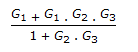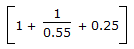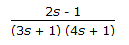Courses

# Test: Instrumentation & Process Control - 5

## 25 Questions MCQ Test Instrumentation and Process Control | Test: Instrumentation & Process Control - 5

Description
This mock test of Test: Instrumentation & Process Control - 5 for Electrical Engineering (EE) helps you for every Electrical Engineering (EE) entrance exam. This contains 25 Multiple Choice Questions for Electrical Engineering (EE) Test: Instrumentation & Process Control - 5 (mcq) to study with solutions a complete question bank. The solved questions answers in this Test: Instrumentation & Process Control - 5 quiz give you a good mix of easy questions and tough questions. Electrical Engineering (EE) students definitely take this Test: Instrumentation & Process Control - 5 exercise for a better result in the exam. You can find other Test: Instrumentation & Process Control - 5 extra questions, long questions & short questions for Electrical Engineering (EE) on EduRev as well by searching above.
QUESTION: 1

Solution:
QUESTION: 2

Solution:
QUESTION: 3

### An amplitude ratio of 0.1 corresponds to __________ decibels.

Solution:
QUESTION: 4

For increased speed of response of an expansion pressure spring thermometer, the thermometer bulb should have a

Solution:
QUESTION: 5

Pressure of 0.0001 absolute psi can be measured by __________ gauge.

Solution:
QUESTION: 6

Pirani gauge is used for the measurement of

Solution:
QUESTION: 7

Transfer functions X/Y for which of the following block diagrams isSolution:
QUESTION: 8

The characteristic equation for the control system with a closed loop transfer function G1/1 + G2 is given by 1 + G2 = 0. The characteristic equation for the control system

Solution:
QUESTION: 9

The __________ of the fluid contained in the temperature sensing element (i.e., bulb) of filled system thermometers changes with change in temperature.

Solution:
QUESTION: 10

__________ thermometer can not measure sub-zero (< 0� C) temperature,

Solution:
QUESTION: 11

Starting temperature of optical radiation pyrometer is __________ �C.

Solution:
QUESTION: 12

Liquid argon level in a pressurised storage tank (at 3 kg/cm2 ) is measured by a/an

Solution:
QUESTION: 13

Which of the systems having following transfer functions is stable?

Solution:
QUESTION: 14

What is the dynamic error in a critically damped second order instrument for a ramp input (At) ?

Solution:
QUESTION: 15

The transfer function for a first order process with time delay is

Solution:
QUESTION: 16

An ideal PID controller has the transfer function. The frequency at which the magnitude ratio of the controller is 1, is

Solution:
QUESTION: 17

The deflection of the free end of the bimetallic strips in a bimetallic thermometer with temperature is nearly

Solution:
QUESTION: 18

Alphatron measures pressure __________ microns.

Solution:
QUESTION: 19

What is the ratio of output amplitude to input amplitude for a sinusoidal forcing function in a first order system ?

Solution:
QUESTION: 20

The unit step response of the transfer functionreaches its final steady state assymptotically after

Solution:
QUESTION: 21

A process is initially at steady state with its output y=l for an input u=l. The input is suddenly changed to 2 at time t=0. The output response is y(t)=1+2t. The transfer function of the process is

Solution:
QUESTION: 22

The term analogous to the electrical current in a thermal system is the

Solution:
QUESTION: 23

Reset rate is the another term used for __________ time.

Solution:
QUESTION: 24

Continuous measurement of moisture in paper is done by

Solution:
QUESTION: 25

Psychrometer determines the

Solution: